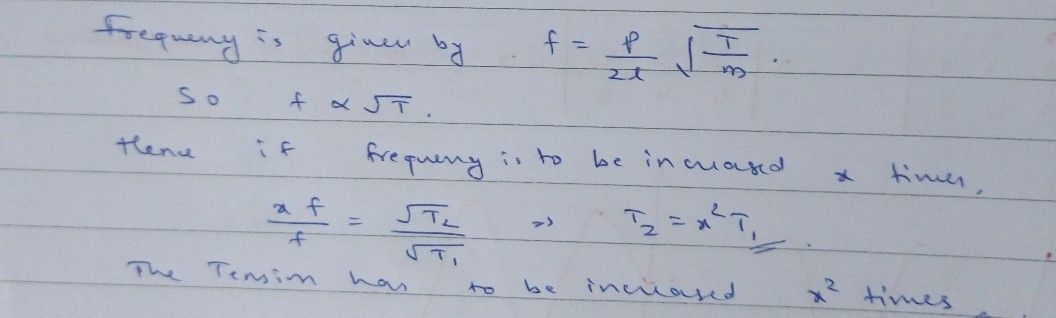Symbol
Problem. To increase the frequency from 100HZ the tension in the string has to be changed $by^{°}$ A. 4times $\infty B_{0}$ $16$ times C. $2$ 2 times D. None of these Page $n%0$